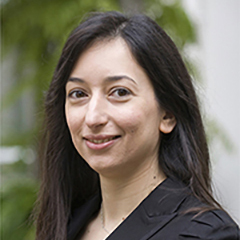# Dr Stella Hadjiantoni

Lecturer in Statistics

## About

`Stella undertook her PhD at Queen Mary, University of London and came to SMSAS in 2016 from a teaching role at LSE.`

## Research interests

`High-dimensional data, large-scale models, computationally intensive problems in statistics, functional data analysis, numerical linear algebra.`

## Publications

### Article

• Hadjiantoni, S. and Kontoghiorghes, E. (2017). A recursive three-stage least squares method for large-scale systems of simultaneous equations. Linear Algebra and its Applications [Online] 536:210-227. Available at: https://doi.org/10.1016/j.laa.2017.08.019.
A new numerical method is proposed that uses the QR decomposition (and its variants) to derive recursively the three-stage least squares (3SLS) estimator of large-scale simultaneous equations models (SEM). The 3SLS estimator is obtained sequentially, once the underlying model is modified, by adding or deleting rows of data. A new theoretical pseudo SEM is developed which has a non positive definite dispersion matrix and is proved to yield the 3SLS estimator that would be derived if the modified SEM was estimated afresh. In addition, the computation of the iterative 3SLS estimator of the updated observations SEM is considered. The new recursive method utilizes efficiently previous computations, exploits sparsity in the pseudo SEM and uses as main computational tool orthogonal and hyperbolic matrix factorizations. This allows the estimation of large-scale SEMs which previously could have been considered computationally infeasible to tackle. Numerical trials have confirmed the effectiveness of the new estimation procedures. The new method is illustrated through a macroeconomic application.
• Hadjiantoni, S. and Kontoghiorghes, E. (2017). Estimating large-scale general linear and seemingly unrelated regressions models after deleting observations. Statistics and Computing [Online]:349-361. Available at: http://dx.doi.org/10.1007/s11222-016-9626-5.
A new numerical method to solve the downdating problem (and variants thereof), namely removing the effect of some observations from the generalized least squares (GLS) estimator of the general linear model (GLM) after it has been estimated, is extensively investigated. It is verified that the solution of the downdated least squares problem can be obtained from the estimation of an equivalent GLM, where the original model is updated with the imaginary deleted observations. This updated GLM has a non positive definite dispersion matrix which comprises complex covariance values and it is proved herein to yield the same normal equations as the downdated model. Additionally, the problem of deleting observations from the seemingly unrelated regressions model is addressed, demonstrating the direct applicability of this method to other multivariate linear models. The algorithms which implement the novel downdating method utilize efficiently the previous computations from the estimation of the original model. As a result, the computational cost is significantly reduced. This shows the great usability potential of the downdating method in computationally intensive problems. The downdating algorithms have been applied to real and synthetic data to illustrate their efficiency.
Last updated